Courses
Courses for Kids
Free study material
Offline Centres
More

# Working of DC Motor, Electric Motor - Principle and Application for JEELast updated date: 05th Dec 2023
Total views: 22.2k
Views today: 1.21k## What is a DC Motor?

A direct current motor (DC motor) is defined as an electrical machine that converts electrical energy into mechanical energy. Based on the definition above, we can conclude that a DC motor is defined as an electric motor that operates on direct current. A DC motor is an electric motor that runs on direct current (DC), unlike an induction motor that operates via an alternating current.

It operates on the Lorentz principle, which states that "a current-carrying conducter placed in a magnetic and electric field experiences a force." That force is known as the Lorentz force . Fleming's Left-hand Rule determines the direction of the mechanical force.

## Working principle or Principle of Operation for DC Motor

The operation of a direct current (DC) motor is based on the principle that when a current-carrying conductor is placed in a magnetic field, the conductor experiences a mechanical force. The direction of force is given by Fleming's left-hand rule and the magnitude of this force is given by:

F= BIL Newtons

Where B is a magnetic field, I is current and L is the length of the conductor.

According to Fleming's Left Hand Rule, if we arrange our thumb, forefinger and middle finger of the left-hand perpendicular to each other, the thumb will point in the direction of the magnetic force, the forefinger will point in the direction of the magnetic field and the middle finger will point in the direction of the current.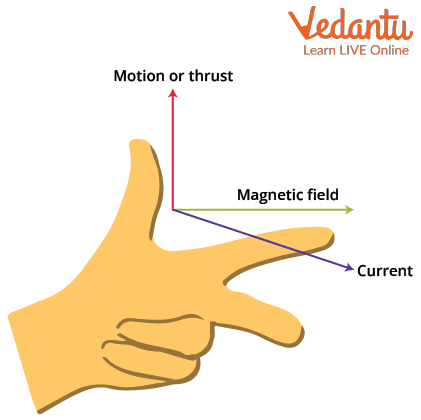Fleming’s Left-Hand Rule

## Basic Motor Operation Function

The field magnets are excited, resulting in the formation of alternate North and South poles.

Currents are carried by armature conductors.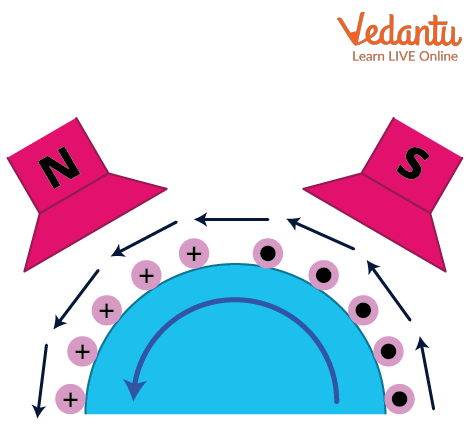Poles and part of DC motor

All North-pole conductors carry currents in one direction, while all South-pole conductors carry currents in the opposite direction. Currents are carried into the plane of the paper by the armature conductors under the N-pole (denoted as in the figure). The conductors beneath the S-pole carry currents away from the plane of the paper (shown in the figure).

A mechanical force acts on each armature conductor because it is carrying current and is placed in the magnetic field. Using Fleming's left-hand rule, it is clear that the force on each conductor tends to rotate the armature anticlockwise. All of these forces combine to create a driving torque that sets the armature to rotate.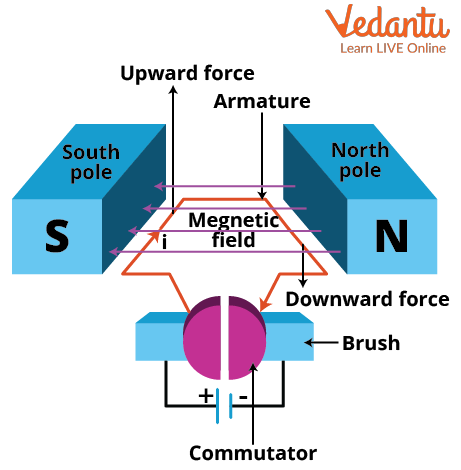Simple DC motor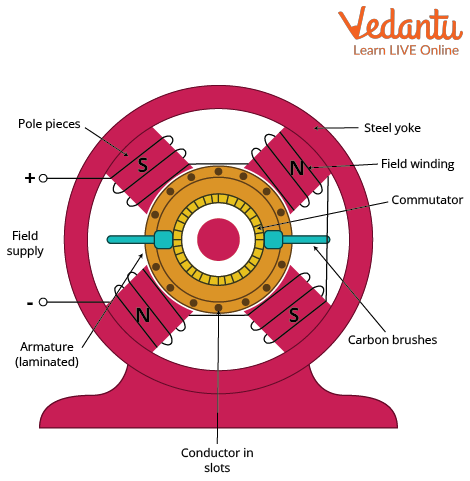Cross-section of DC motor

The current in a conductor is reversed when it moves from one side of a brush to the other. At the same time, it is influenced by the next pole, which has the opposite polarity. As a result, the direction of the force on the conductor remains constant. It should be noted that the function of a commutator in a motor is the same as that of a commutator in a generator. It helps to develop a continuous and uni-directional torque by reversing the current in each conductor as it passes from one pole to another.

## Application of DC Motor

A direct current motor is a type of motor in which we use a permanent magnet to generate the necessary magnetic field. As this motor does not need to control the speed, it is used in applications like:

• Washer

• Automobiles as a starter motor

• Personal computer disc drives

• Toys

• Wheelchairs

• Blowers in heater and air conditioners.

## Working Principle of Electric Motor

The electric motor operates on the principle that when a coil is placed in a magnetic field, current flows through it, causing the coil to rotate.

Take two bar magnets and align the poles with a small space between them. Make a loop with a small length of conducting wire. Maintain this loop in the space between the magnets so that it remains within the magnets' sphere of influence. Now for the final section, join the loop's ends together.

When you pass electricity through your simple circuit, you will notice that your loop ‘moves’. What causes this? The magnetic field produced by the magnets interferes with the magnetic field produced by the electric current flowing in the conductor. As the loop has become a magnet, one side will be drawn to the north pole and the other to the south pole. This causes the loop to rotate indefinitely. This is the working principle of an electric motor.

## Working of Electric Motor

The electrical motor is a device that converts electrical energy into mechanical energy. In simple terms, the electrical motor is a device used for producing rotational energy.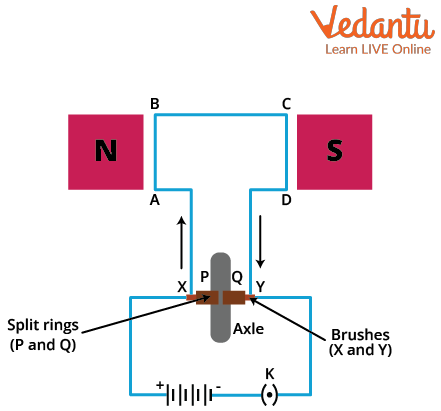Electric motor

According to the diagram, the current enters the coil ABCD from brush X. ABCD is a current-carrying coil that is placed in a magnetic field that is perpendicular to it. So, using Fleming's left-hand rule, we can say that AB moves downward and CD moves upward. CD moves towards the north pole in the next half rotation, while AB moves towards the south pole. Using Fleming's left-hand rule again, we can see that AB moves upward and CD moves downward. This process continues and the coil begins to rotate.

## Conclusion

In short, an electrical DC motor is an electrical machine that converts electrical energy into mechanical energy. Therefore, we have discussed how DC and electric motors work and their applications. We have learnt the working principle of the DC and electrical motor and also the rules used to identify the direction of the force produced. This article gives a detailed description of the DC electric motor so that you will have a better understanding of the concept.

## FAQs on Working of DC Motor, Electric Motor - Principle and Application for JEE

1. How do you reverse the rotational direction of a D.C. Motor?

We can reverse the rotational direction of a direct current motor by reversing the field or armature current. When both currents are reversed, the motor will run in the opposite direction. Another method is by inverting the polarity of the applied armature voltage by armature leads reversing their direction.  In this case also the same as the above case will happen when the voltage is reversed, so the motor will run in the opposite direction. This works with shunt, series and compound wound DC motors.

2.  What Exactly Is Speed Regulation?

Speed regulation is the change in speed caused by reducing the load on the motor from its rated value to zero. We can also define it the other way, as the change in the speed from full-load to no-load and is expressed as a percentage of the full-load speed.

• It is given as a percentage of the rated load speed.

• Thus, the factors affecting the speed of a DC motor are

1. The flux  $\phi$ -Changing  $\phi$ by controlling the current through the field winding.

2. The voltage across the armature- changing the armature path resistance will change the voltage applied across the armature.

3. The applied voltage V- changing the applied voltage is called the ‘voltage control method’.